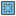• # No idea how to improve the speed

## Question related to missionMagic Square

Hi: I write my own code which passed the standard tests, but take forever to pass the one on website. I'll really appreciate it if you have any suggestion on how to improve the speed. Thanks! Here's my code:

```import numpy as np
from copy import copy
from itertools import permutations

def checkio(data):
square = np.array(data)
n = len(data)
s = int(n*(1+n**2)/2)
templist = []
coord = np.where(square == 0)

def check(square):
temp1 = np.sum(square, axis=1)
temp2 = np.sum(square.T, axis=1)
temp3 = np.sum(np.diagonal(square))
temp4 = np.sum(np.diagonal(square[::-1]))
if temp3 != s or temp4 != s:
return False
if np.any(s != temp1) or np.any(s != temp2):
return False
return True

for i in range(1,n**2+1):
if i not in square:
templist.append(i)

for i in permutations(templist):
squarecopy = copy(square)
count = 0
for j in zip(coord, coord):
squarecopy[j] = i[count]
count += 1
if check(squarecopy):
return squarecopy.tolist()
```27